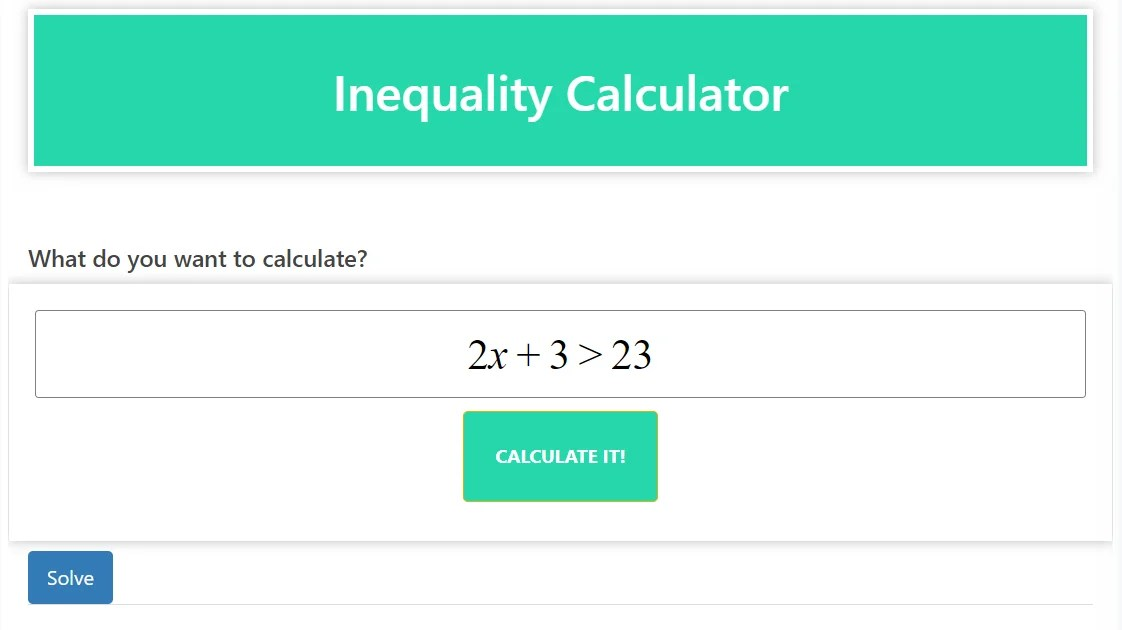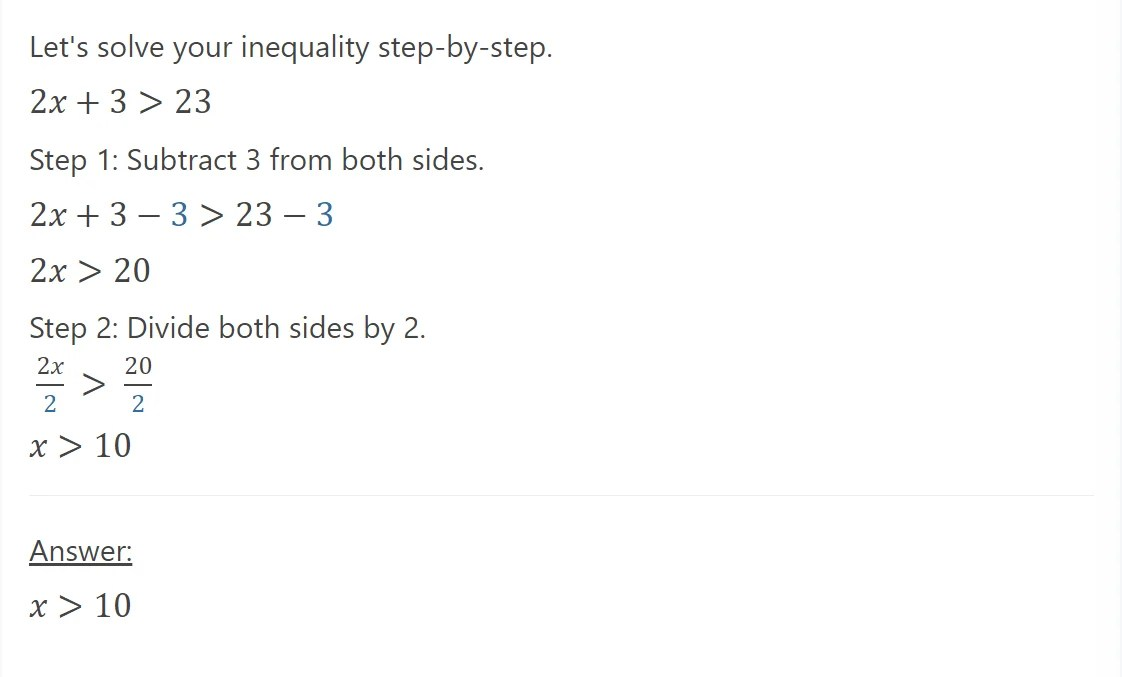# Inequality Calculator – Your Reliable Math Solver for Inequalities

### Introduction:

Dealing with inequalities in math can be challenging, but don’t worry! Our inequality calculator is here to simplify the process. This powerful math solver enables you to easily analyze and solve inequalities, giving you accurate results and a deeper understanding of mathematical concepts.

### Explanation:

Inequality Calculator is a valuable tool for solving and understanding inequalities. In mathematics, inequalities express a relationship between two values that are not necessarily equal. They are usually represented using symbols such as “<” (less than), “>” (greater than), “<=” (less than or equal to), “>=” (greater than or equal to), and ” is represented. !=” (not equal).

Our calculator provides a comprehensive set of features for handling different types of inequalities including linear inequalities, quadratic inequalities, and absolute value inequalities. Here’s how our inequality calculator can help you:

### Inequality Solution:

The calculator allows you to input an inequality and provides step-by-step solutions. It guides you through the process of solving inequality, helping you understand the methods and logic behind each step.

### graphical representation:

Viewing inequalities on a graph can be helpful in understanding their solutions. Our calculator can plot the inequality on a coordinate plane, allowing you to analyze solution sets and identify regions that satisfy the inequality.

### interval notation:

In addition to graphical representation, the calculator can present solutions using interval notation. This notation provides a concise way of representing the range of values satisfying the inequality, making it easier to interpret and work with.

### Inequality Operation:

The inequality Calculator supports various operations on inequalities, such as adding, subtracting, multiplying, and dividing both sides of an inequality. It applies proper rules to maintain the integrity of the inequality and find the correct solution.

By using our Inequality Calculator, you can save time and increase your understanding of inequalities. It provides a structured approach to solving inequalities and provides valuable insight into the relationships between values. Inequality Calculator (Maths solver)

### conclusion:

Simplify your journey through inequalities with our inequality calculator. This reliable maths solver empowers you to analyze and solve different types of inequalities, providing step-by-step solutions, graphical representation, and interval notation. Experience the convenience and effectiveness of our inequality calculator today, and conquer inequalities with confidence. Inequality Calculator (Maths solver)